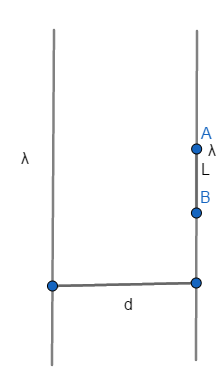# Two long thin charged rods with charge density lambda each are placed parallel to each other at a...

## Question:

Two long thin charged rods with charge density lambda each are placed parallel to each other at a distance d apart. The force per unit length exerted on one rod by the other will be?

## Electric Field

The electric field due to an infinite line of charge having uniform linear charge density at a distance from the line is given by

{eq}\begin{align} E = \frac{2k \lambda}{r} \end{align} {/eq}

Where {eq}\lambda {/eq} is the linear charge density, and r is the distance of the point of observation to the center of the charged rod.

• Charge density of each rod is {eq}\lambda {/eq}
• Distance between the rods is {eq}d {/eq}

Let us draw a diagram of the situationLet us consider a segment AB of length L on the second rod, now the total charge on this segment of the rod is

{eq}\begin{align} q = \lambda L \end{align} {/eq}

Now the electric field E due to charged rod one at the location of rod B

{eq}\begin{align} E = \frac{2k \lambda}{d} \end{align} {/eq}

Now force on the segment AB due to charges rod one, considering the segment AB as point charge as size of the segment is negligible as comapred to the length of rod one

{eq}\begin{align} F = qE \end{align} {/eq}

{eq}\begin{align} F = \frac{2k \lambda.q}{d} \end{align} {/eq}

{eq}\begin{align} F = \frac{2k \lambda. \lambda L}{r} \end{align} {/eq}

Now force per unit length

{eq}\begin{align} f = \frac{F}{L} \end{align} {/eq}

{eq}\begin{align} \color{blue}{\boxed{ \ f = \frac{2k \lambda^2}{r} \ }} \end{align} {/eq}